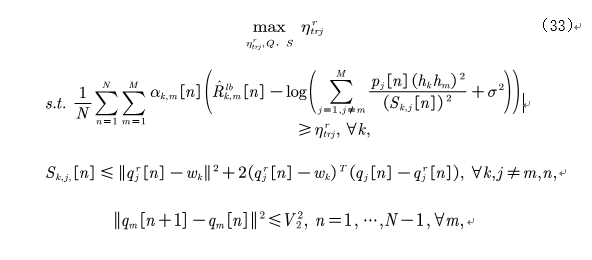# About CVX Illegal operation: log( {convex} )

Part of convex function is as follows, and it’s convexI try to use CVX to solve this problem, but the solver always failed.
and this is my code:
function [q1,q2,optval_traj] = Trajectory(a1,a2,p1,p2,q1_pre,q2_pre,w)

global K N T noise H_min H_max v_max v_min d_min h_k h_m;

V1 = v_minT/N;
V2 = v_max
T/N;

d1=zeros(K,N);
d2=zeros(K,N);
for k=1:K
d1(k,:)=sum_square_abs(q1_pre-w(:,:,k));
d2(k,:)=sum_square_abs(q2_pre-w(:,:,k));
end

temp=ones(K,1)p1(h_kh_m)^2./d1.^2+ones(K,1)p2(h_kh_m)^2./d2.^2;
I1=2ones(K,1)p1(h_kh_m)^2./(log(2)d1.^3.(temp+noise));
I2=2ones(K,1)p2(h_kh_m)^2./(log(2)d2.^3.(temp+noise));

cvx_begin
cvx_solver Mosek
variable R_traj
variable q1(3,N) nonnegative
variable q2(3,N) nonnegative
variable S1(K,N)
variable S2(K,N)
expression g1(K,N)
expression g2(K,N)
expression R1_lb(K,N)
expression R2_lb(K,N)
expression sum(K,N)
expression t1(K,N)
expression t2(K,N)

    sum=ones(K,1)*p1*(h_k*h_m)^2./d1.^2+ones(K,1)*p2*(h_k*h_m)^2./d2.^2;

R1_lb=-I1.*S1-I2.*S2+log(sum+noise)/log(2);
R2_lb=-I1.*S1-I2.*S2+log(sum+noise)/log(2);

for k=1:K
for n=1:N
t1(k,n)=(q1_pre(:,n)-w(:,n,k))'*(q1(:,n)-q1_pre(:,n));
t2(k,n)=(q2_pre(:,n)-w(:,n,k))'*(q2(:,n)-q2_pre(:,n));
end
end

maximize (R_traj)
subject to

sum(a1.*(R1_lb-log(ones(K,1)*p2*(h_k*h_m)^2.*inv_pos(S2)+noise)/log(2))+...
a2.*(R2_lb-log(ones(K,1)*p1*(h_k*h_m)^2.*inv_pos(S1)+noise)/log(2)),2) >= N*R_traj;
S1 <= d1+2*t1;
S2 <= d2+2*t2;
for n=1:N-1
sum_square_abs(q1(:,n+1)-q1(:,n)) <= V2^2;
sum_square_abs(q2(:,n+1)-q2(:,n)) <= V2^2;
end
for n=1:N-1
sum_square_abs(q1_pre(:,n+1)-q1_pre(:,n))+2*sum((q1_pre(:,n+1)-q1_pre(:,n)).*(q1(:,n+1)-q1(:,n))) >= V1^2;
sum_square_abs(q2_pre(:,n+1)-q2_pre(:,n))+2*sum((q2_pre(:,n+1)-q2_pre(:,n)).*(q2(:,n+1)-q2(:,n))) >= V1^2;
end
-sum_square_abs(q1_pre-q2_pre)+2*sum((q1_pre-q2_pre).*(q1-q2)) >= d_min^2;
q1(:,1) == q1(:,N);
q2(:,1) == q2(:,N);
for n=1:N
H_min <= q1(3,n) <= H_max;
H_min <= q2(3,n) <= H_max;
end


cvx_end

optval_traj = cvx_optval;
end
I have tried many many methods, but still cannot solve it, so how to express the problem properly in CVX?
I really appreciate your generous help.

1 Like

\begin{array}{l} \log \left( {1 + \frac{C}{{{x^a}}}} \right) \le D{\rm{ \ can\ be\ expressed\ as}}\\ \left\{ \begin{array}{l} \log \left( {1 + {e^z}} \right) \le D{\rm{ }}\quad \to\quad {\rm{ log\_sum\_exp([0\ z])}} \le {\rm{D}}\\ \log C - a\log x \le z \quad \to\quad {\rm{ log(C) - a*log(x)}} \le z \end{array} \right. \end{array}

1 Like

Jack, I appreciate your reply. But I don’t know how to use this formulation in my case. Could you please explain it more clearly? Thank you very very much!

You were asking how to express log(ones(K,1)*p2*(h_k*h_m)^2.*inv_pos(S2)+noise)/log(2) in your code. Here’s how:Define 2 new variable z and D , then replace log(ones(K,1)*p2*(h_k*h_m)^2.*inv_pos(S2)+noise)/log(2) with D, and add the inequalities I showed you above, which you will find they’re equivalent mathematically. About the use of log_sum_exp, see

About modeling (the process above) ,see

Hi, Jack. Thank you so much for your advice. I followed your advice and modified my code. I define D as a matrix and z as a scalar. This part of code is as following
maximize (R_traj)
subject to
log_sum_exp(z1) <= D1;
log(ones(K,1)p2(h_kh_m)^2)./log(2)-2log(S1)./log(2) <= z1;
log_sum_exp(z2) <= D2;
log(ones(K,1)p1(h_kh_m)^2)./log(2)-2log(S2)./log(2) <= z2;
sum(a1.(R1_lb-D1)+a2.(R2_lb-D2),2) >= N*R_traj;
But when I try to run my code, I meet another error

sum(a1.(R1_lb-D1)+a2.(R2_lb-D2),2) >= N*R_traj;
I don’t know how to handle this error. Could you please tell me more about it? Thank you vvvvery much!

There must be some bug in your code. Its hard to guess because the information you provide is too little, and you did not format your code in a Mardown format. And, please translate the errors into English so the experts can read them.

sorry i edit my previous comment, there was a mistake in the inequality of log_sum_exp,pls read them again.

Thank you very much Jack. I edit my code according to your reply. There was another error

Illegal operation: {complex constant} - {concave}

the code is as follows
log(ones(K,1)*p2*(h_k*h_m)^2)./log(2)-2*log(S1)./log(2) <= z1;
What can I do to solver this problem?
The error says {complex constant} - {concave}, therefore obviously the log(ones(K,1)*p2*(h_k*h_m)^2)./log(2) is the {complex constant}. So I guess ones(K,1)*p2*(h_k*h_m)^2 is <=0, which makes the log of it become a complex constant.